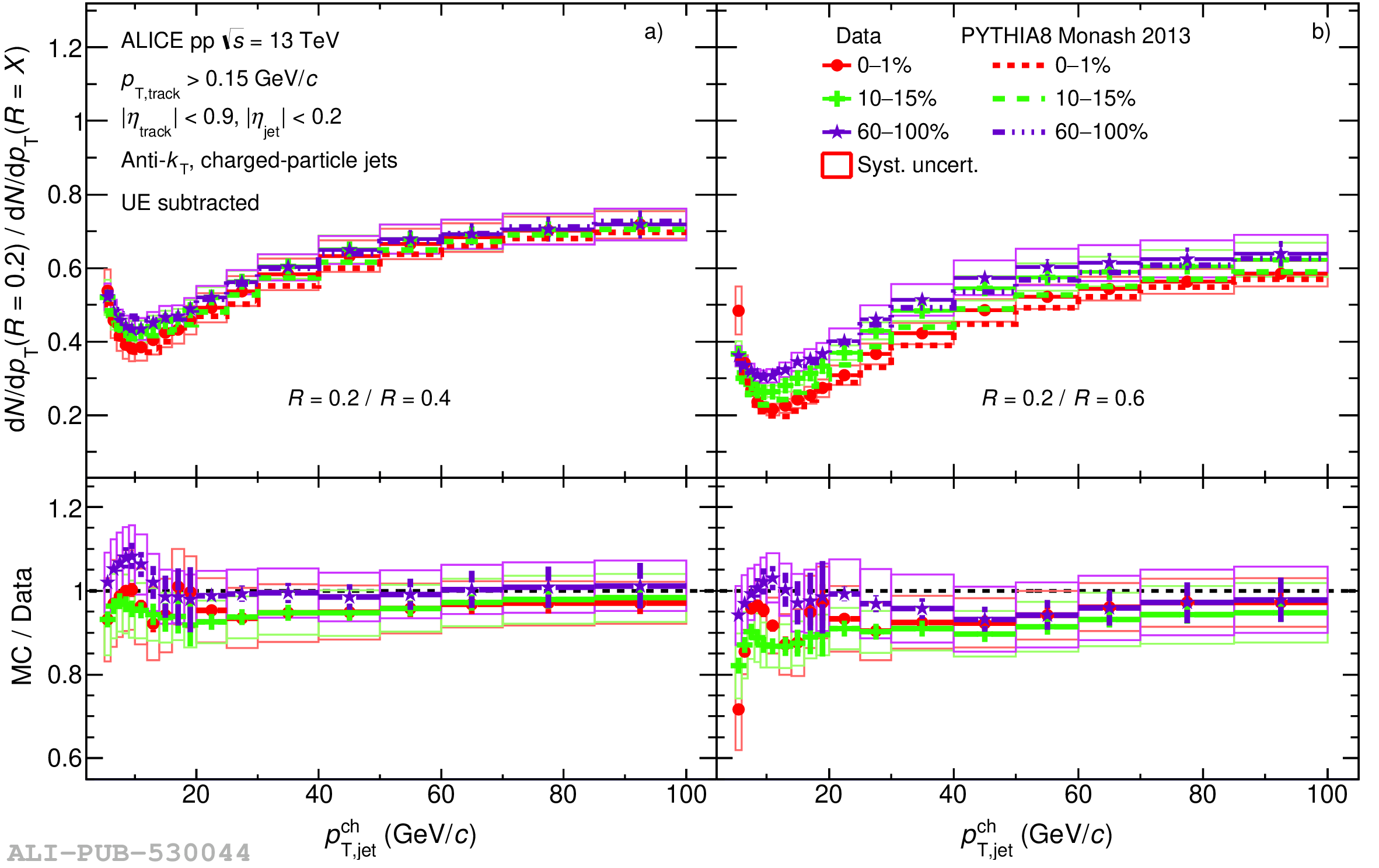# Figure A.6

 Comparison of jet production ratios of $R = 0.2$ to a) $R = 0.4$, b) $R = 0.6$ in three different multiplicity classes and compared with PYTHIA MC simulations. Statistical and the total systematic uncertainties are shown as vertical error bars and boxes around the data points, respectively.